RUSSIAN JOURNAL OF EARTH SCIENCES, VOL. 16, ES6003, doi:10.2205/2016ES000585, 2016

# Growth of the inner core in the mean-field dynamo model

M. Yu. Reshetnyak1,2

1Schmidt Institute of Physics of the Earth of the Russian Academy of Sciences, Moscow, Russia

2Pushkov Institute of Terrestrial Magnetism, Ionosphere and Radio Wave Propagation of the Russian Academy of Sciences, Moscow, Russia

### Abstract

Application of Parker's dynamo model to the geodynamo with the growing inner core is considered. It is shown that decrease of the inner core size, where intensive magnetic field generation takes place, leads to the multi-polar magnetic field in the past. This effect reflects the decrease of the region of the effective magnetic field generation. The process is accompanied by increase of the reversals number and decrease of intensity of the geomagnetic field. The constraints on the mechanisms of convection in the liquid core are discussed.

### Introduction

Geomagnetic field, generated by the dynamo process in the liquid core of the Earth, is a unique source of information on the internal structure of the planet. Due to low conductivity of the mantle, magnetic field penetrates from the surface of the inner core to the surface of the planet without significant distortions. The age of the magnetic field, estimated as 3.5Gy [Tarduno at al., 2010], see also review [Reshetnyak and Pavlov, 2016], is compared to the age of the Earth itself 4.5Gy. So far during the "magnetic" epoch the liquid and solid cores of the Earth evolved, it is tempting to detect variations of the geomagnetic field, concerned with the evolution of the planet. The main reason of such variations is the formation of the inner core, which effects the dynamo process at least in two ways.

Firstly, the growth of the inner core can influence on the magnetic field generation through the pure geometrical factor, which has no relation to the physical mechanisms of convection. The growth of the inner core leads to decrease of the liquid core, and to increase of the radius of the Taylor cylinder (the imaginary cylinder elongated along the axis of rotation and surrounding the inner core). So far the geomagnetic dipole between the reversals wanders inside of the cylinder, one can expect increase of the virtual geomagnetic pole fluctuations relative to the axis of rotation during the evolution of the Earth.

The other reason is existence of two mechanisms of convection simultaneously: the thermal convection, concerned with the radioactive heating, and compositional convection, which is associated with the growth of the inner core. The latter mechanism is supposed to be more efficient, because the heat is injected at the bottom of the liquid core. Compositional convection can produce three times more energy than the thermal convection. In the thermal convection the radioactive heat sources are distributed smoothly in the bulk of the liquid core. It is quiet different from the compositional convection, where latent heat sources, concerned with crystallization process at the surface of the inner core, are localized at the inner core boundary. The shift of this boundary, where maximum of the magnetic field generation is observed in 3D models, relative to the observer at the surface of the planet, can change spatial spectrum of the observable magnetic field.

These more or less evident assumptions were already tested in some 3D models, however the obtained conclusions still can not provide the robust scenario of the core evolution, supported by the paleomagnetic measurements [Reshetnyak and Pavlov, 2016]. There are at least two reasons of such failure.

The first one is that geomagnetic field indeed does not reveal significant changes during the supposed period of the inner core formation 1-2Ga. The other point is that 3D models, due to its complexity, provide too short time series, which are not sufficient for the evolutionary processes treatment. Moreover, having deal with the 3D models, one substitutes the black box of the MHD system in the core, with the another one, named the non-linear system of 3D partial differential equations, which can be solved only numerically. Note that the parameters used in the models in its turn are very far from that ones in the core. Even if the model corresponds to observations, the level of understanding of the physics of such a complex system is not satisfactory. Interpretation of the dynamo process using the simpler and more obvious scenarios is appreciated.

It motivates us to use further the simpler, 2D Parker's dynamo model [Parker, 1955], which was developed latter to the mean-field dynamo theory [Krause and Rädler, 1980]. The energy sources: the $\alpha$-effect and differential rotation, are taken from 3D simulations, and can vary with change of the inner core's size in the prescribed manner. It is also considered the different spatial distributions of the $\alpha$-effect and differential rotation, which depend on the intensity of the heat sources in the core. As a result we present dependence of the observed at the surface of the core magnetic field on the radius of the solid core and analyze how the spatial spectrum of the magnetic field varies during the Earth's evolution. This analysis helps to distinguish the main features of the flows in the liquid core responsible for generation of the dominant dipole magnetic field in the past.

### Dynamo Model

The mean magnetic field $\mathbf{B}$ is governed by the induction equation

\begin{equation} \tag*{(1)} \displaystyle {\partial\mathbf{B}\over\partial t} = \nabla \times \Big( \alpha\,\mathbf{B}+\mathbf{V}\times \mathbf{B} -\eta\, {\rm rot}\mathbf{B} \Big), \end{equation}

where $\mathbf{V}$ is the large-scale velocity field, $\alpha$ is the $\alpha$-effect, and $\eta$ is a magnetic diffusion. The magnetic field ${\mathbf{B}}=\left( {\mathbf{B^p}},\, {\mathbf{B^t}} \right)$ has two parts: the poloidal component ${\mathbf{B^p}}=\nabla\times \mathbf{A}$, where $\mathbf{A}$ is the vector potential of the magnetic field, and the toroidal component $\mathbf{B^t}$.

In the axi-symmetrical case the vector potential $\mathbf{A}$ and $\mathbf{B^t}$ have the only one azimuthal component in the spherical system of coordinates $(r,\,\theta,\, \varphi)$: ${\mathbf{A}}(r,\,\theta)=(0,\, 0,\, A)$, and $\mathbf{B^t}(r,\,\theta)=(0,\, 0,\, B)$.

The poloidal field can be written in the form:

\begin{equation} \tag*{(2)} \displaystyle {\mathbf{B^p}}= \left( {1\over r\, \sin\theta}{\partial\over\partial \theta }\left( A\, \sin\theta \right),\, -{1\over r} {\partial \over \partial r} \left( r\, A \right),\, 0 \right). \end{equation}

In terms of scalars $A$ and $B$ Eq(1) is reduced to the following system of equations:

\begin{eqnarray*} \displaystyle {\partial{ A}\over\partial t}=\alpha {B} + \left(\mathbf{V}\times\,\mathbf{B}\right)_\varphi +\eta \left( \nabla^2 - {1\over r^2\sin^2\theta} \right) {A} \end{eqnarray*}

\begin{equation} \tag*{(3)} \displaystyle {\partial{B}\over\partial t}={\rm rot}_\varphi \left( \alpha\,\mathbf{B} + \mathbf{V}\times\,\mathbf{B}\right)+\eta \left( \nabla^2 -{1\over r^2\sin^2\theta} \right){B}, \end{equation}

where the subscript $\varphi$ corresponds to the azimuthal component of the vector, and $\eta$ is equal to unity.

Eqs(3), solved in the spherical shell $r_c\le r\le r_\circ$ with variable $r_c$, and $r_\circ=1$, are closed with the pseudo-vacuum boundary conditions: ${ B}=0$, and $\displaystyle {\partial \over\partial r} \left( r A\right)=0$ at $r_c$, and $r_\circ$. The fields are vanishing at the axis of rotation $\theta=0,\, \pi:$ $A=B=0$. The simplified form of the vacuum boundary condition for $A$ is well adopted in dynamo community, and presents a good approximation of the boundary with the non-conductive medium [Jouve et al., 2008]. The reason why the vacuum boundary condition is used at the inner core boundary is concerned with the weak influence of the inner core on the reversals statistics of the magnetic field [Wicht, 2002].

In the general case velocity $\mathbf{V}$ is a three-dimensional vector, which depends on $r$ and $\theta$. Further we consider only the effect of the differential rotation, concerned with the $\varphi$-component of $\mathbf{V}$, leaving the input of the meridional circulation $(V_r,\,V_\theta)$ out of the scope of the paper. The amplitude of the azimuthal velocity component $V_\varphi=\Omega s$, where $\Omega$ is the angular velocity of the fluid, and $s$ is the distance from the axis of rotation $\mathbf{z}$, is defined by constant $C_\omega$.

The model is closed by the $\alpha$-quenching in the local algebraic form:

\begin{equation} \tag*{(4)}\displaystyle \alpha=C_\alpha\,{\alpha_\circ \over 1+E_m(r,\,\theta)}, \end{equation}

where $E_m$ is the magnetic energy, and $C_\alpha$ is a constant.

The system (3),(4) was solved using the $4^{th}$-order Runge-Kutta method, where spatial derivatives in the r.h.s. were approximated by the second-order central-differences at the mesh grid $(r,\, \theta)$ $(101\times 101)$. These algorithms resulted in C++ object oriented code with OpenMP for parallelization. The post-processor graphic visualization was organized using the Python graphic library MatPlotlib. All simulations were done under Ubuntu OS. See the details of the benchmark [Jouve et al., 2008] in [Reshetnyak, 2014].

To demonstrate dependence of solution of Eqs(3),(4) on the different parameters the MPI wrapper was used to run the main program at two cluster supercomputers: Lomonosov in Moscow State University and at the Joint Supercomputer Center of RAS. The wrapper called the main program with the fixed different values of parameters, like radius of the inner core $r_c$, and amplitudes of the $\alpha$- and $\omega$-effects, $C_\alpha$, $C_\omega$, at the different processors and then gathered all the data at the end of simulations.

### Spatial Distribution of the Fields in Presence of the Rapid Rotation

In the first approximation effect of rotation results in elongation of all the fields along the axis of rotation. The linear theory predicts that derivatives of the velocity and temperature fluctuation fields along the axis of rotation is five orders of magnitude smaller than in the perpendicular plane [Roberts, 1968; Busse, 1970]. The turbulent effects decrease this difference, leaving it however still substantial. This feature distinguishes the planetary dynamo from the dynamo in the galaxies and in the majority of the stars, where rotation is not so strong. It means that in the considered 2D model gradients of the prescribed $\alpha_\circ$ and $\Omega$ should also reflect this feature.

This point is very tricky, because the mean-field approach is based on existence of the intermediate scale, concerned with the averaging of the turbulent fields. This averaging leads to the opposite effect, concerned with smoothing of the sharp gradients of the velocity and temperature fluctuations fields, which are indeed observed in 3D dynamo models. As a result the difference between the derivatives of the fields in $s$- and $z$-directions in the cylindrical system of coordinate should substantially decrease.

Having these arguments in mind, only the large-scale features of the flow, taken from 3D simulations, should be included in the mean-field dynamo models. For the angular velocity $\Omega$ it is dependence only on $s$-coordinate, so that for large $s$ $\Omega>0$ and for small $s$ $\Omega$ is negative, see, e.g. [Busse and Simitev, 2008]. As regards to the distribution of $\alpha$ it should change sign at the equator plane and concentrate near the Taylor cylinder, where cyclonic convection is localized [Reshetnyak, 2012].

We start from the more explored to the moment in the geodynamo regime with the present size of the solid core $r_c=0.35$, and introduce the following proxies to the $\alpha_\circ$- and $\Omega$-distributions:

\begin{eqnarray*} \alpha_0^I=\widehat{C}_\alpha(1-erf(1.25|z|))e^{-S_c (s - 1.1r_c)^2}s\cos(\theta), \end{eqnarray*}

\begin{equation} \tag*{(5)} \Omega^I= -\widehat{C}_\Omega \left(1+erf(8(s-r_c))\right) \cos\left({\pi(s-r_c)\over 1-r_c}\right), \end{equation}

with $S_c=67$, $s=r \sin(\theta)$, and $z=r \cos(\theta)$. The positive constants $\widehat{C}_\alpha$, $\widehat{C}_\Omega$ satisfy conditions: the maximal values of $|\alpha_0^I|$, $|\Omega^I|$ are equal to unity.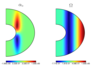Figure 1

These distributions, Figure 1, correspond to the strongly geostrophic state near the threshold of convection generation (small Rayleigh number), where convection is concentrated outside of the Taylor cylinder. It appears [Reshetnyak, 2014] that using these distributions one can generate the Earth-like magnetic field that resembles the well-known $Z$-field distributions in Bragisnky's geodynamo model [Braginsky, 1975].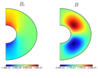Figure 2

It is interesting that $B_r$-component is concentrated inside of the cylinder, Figure 2, where $\alpha_\circ$ is small. This is the essentially the non-linear effect, concerned with the small quenching effect inside of the cylinder and near the inner core boundary. The total magnetic energy $E_m$ with the main contribution from the toroidal field counterpart (see distribution of $B$-component in Figure 2) is smaller inside of the cylinder, and equal to zero at the boundary due to the boundary conditions. Then, following Eq.(4) one has smaller $\alpha$-quenching in these regions.

On the contrary, the large toroidal magnetic field outside of the cylinder sweeps out $\alpha$ from the region, suppressing the poloidal field generation.

In other words the specific of our model is that the maximum of the poloidal magnetic field is determined by the intensity of the $\alpha$-quenching rather than by the amplitude of the original $\alpha_\circ$.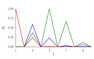Figure 3

If we believe that distributions of $\alpha_\circ$ and $\Omega$ follow location of the Taylor cylinder then we can extrapolate distributions (5) to the smaller $r_c$. However this approach leads to contradiction with the pillar of paleomagnetism that the geomagnetic field should be dipole. This is demonstrated in Figure 3, where the gradual increase of the higher harmonics strength in the Mauersberger spectrum ${\cal S}(l)$ with decrease of $r_c$ is clearly observed.

The decrease of the dipole is closely related to the geostrophic balance in the core. In presence of the geostrophic balance: the balance of the Coriois force and pressure gradient, the velocity and temperature fields variations are elongated along the axis of rotation. Moreover, the magnetic field also "feels" the geograpical poles, so that the geomagnetic dipole, as already was mentioned, prefers to stay inside of the Taylor cylinder. The decrease of the radius of the Taylor cylinder decreases the scale of the magnetic field, concentrated inside of the cylinder. As a result the dipole contribution to the spectrum becomes smaller. This exactly what we observe in Figure 3.

It is worthy to note that this effect is stronger than the opposite effect, concerned with the radial decay of the magnetic field: the smaller is the inner core, the larger is the distance from the surface of the core to the inner core boundary, where the magnetic field is strong. Then the ratio of the dipole component to the other harmonics at the Earth's surface will increase with increase of the liquid core thickness. However, as we have just shown, the effect, concerned with geostrophy, is stronger than the effect, concerned with the radial decay of harmonics.

There are two ways out of this situation. Of course, it would be naive to expect now from the paleomagnetic community estimates of evolution of the Mauersberger spectrum on the geological times. That is why we can not exclude the enforce of the higher harmonics in the spectrum in the past at all. However rejection of the dipole hypothesis of the paleo field leads to the principal impossibility of any useful for theoreticians mathematical description of the fields spatial structure. Then it is more instructive to consider how our model can be modified to adjust the dipole hypothesis in the past.

Firstly we have to note that we considered the differential rotation which does not depend on $z$ at all. This flow corresponds to the regime with the very small Ekman numbers $\rm E$, which is indeed expected in the inner core: ${\rm E} \sim 10^{-16}$. The increase of $\rm E$ breaks geostrophy and can lead to increase of the flowing up to the surface of the liquid core magnetic field. In other words, dependence on $s$-coordinate will change to dependence on $r$. This effect can be observed in 3D dynamo models with moderate Ekman numbers. Recall that in majority of the dynamo models $\rm E$ is in the range $10^{-6}\div 10^{-4}$ that is still quite far from the geophysical values in the liquid core. In this sense the prescribed geostrophical $\Omega$ in our 2D model can be more realistic. It is important that for the compositional convection, where the heat flux, associated with crystallization, is injected at the inner core boundary. Then, in presence of the geostrophic state, decrease of $r_c$ leads to the decrease of the dipole field contribution. And on contrary, when the radial Archimedean force is quite strong in the thermal convection models, the magnetic field expands to the surface of the liquid core, increasing the scale. In this case the dipole field can be quite strong at the surface of the liquid core even at the small $r_c$.

Situation is even worth if we take into account that increase of the heat sources leads to the shift of convection from the part of the liquid core outside of the Taylor cylinder to its inner part [Glatzmaier and Roberts, 1995]. If for the present size of the inner core such convection still generates the dipole field, then for the smaller value of $r_c$ there is no chance for the dipole magnetic field. The same is for the thermal convection with the prescribed temperatures at the inner core and mantle boundaries, where the density of the heat flux $q\sim 1/r$. For this model generation of the magnetic field will be more effective near the inner core boundary, where $q$ is larger.

Summing up, we have that localization of the magnetic filed generation near the liquid core in presence of the geostrophic balance leads to decrease of the spatial scale of the magnetic field at the liquid core surface. It means that thermal convection with the radioactive heating suites better to the dipole filed generation in the past than the compositional convection, or the thermal convection model with the fixed temperatures at the boundaries.

We note that only taking into account of the inner core evolution allows to come to such a conclusion and reject some models, which give similar configurations of the magnetic field at the surface of the Earth for the present value of the inner core radius $r_c$.

Besides the mentioned above increase of the scale, produced by the radioactive heating, there is the another physical effect, which leads to the same result. The majority of the modern 3D dynamo models is based on the Boussinesq approximation of convection. It means that effect of compressibility is taken into account only for derivation of the Archimedean forces. Then, the kinetic helicity, $\chi=\mathbf{V}\cdot{\rm rot}\mathbf{V}$, closely connected to the $\alpha$-effect, is generated near the boundaries of the liquid core, and in the vicinity of the Taylor cylinder. For the realistic values of Ekman number the scales of the boundary layers, where helicity of the incompressible fluid is generated, are too small to generate the magnetic field. It means that extrapolation of helicity profiles to the realistic $\rm E$ can lead to the break of the magnetic field generation at all.

However, in the compressible fluid kinetic helicity can generate in the bulk of the volume due to expansion/compression of the rotating flowing up/down fluid particle. This effect leads to increase of the $\alpha$-effect spatial scale. The drop of the density $\rho$ from the inner core boundary to the core-mantle boundary is 20%. This is quiet enough for discernible contribution to the total $\alpha$-effect [Reshetnyak, 2012]. Note that this effect has no connection to the inner core at all. In another words, we assume that for the state with the small inner core, where the drop of $\rho$ is even larger than for the present time, effects of compressibility should be taken into account to provide generation of the large-scale magnetic field in Parker's dynamo model.

Fortunately, having deal with the mean-field dynamo model we can drop out some details of the flow on the scales, including the inner core size in the past, leaving the geostrophy of the flow as the main feature. Further we disconnect the localization of the $\alpha$-effect and differential rotation from the boundary of the Taylor cylinder. Then, growth of the inner core will lead only to the increase of the bulk of the core, but will not change substantially distributions of the energy sources in the model. Such assumptions are valid to the compressible fluid and thermal convection with distributed over the liquid core heat sources.

To explore this possibility we consider the smoother distribution of $\alpha_\circ$, see Eqs(5), with $S_c=17$, and fixed $r_c=0.35$, even if the volume of the liquid core in Eqs(3) was changed. For the angular velocity $\Omega$ we used the following dependence $\Omega^{II}= -C_\Omega\cos(\pi s)$, where we kept geostrophy, i.e. dependence on $s$ coordinate only, and the change of $\Omega$ sign from negative to positive with increase of $s$.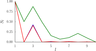Figure 4

These assumptions let to generate the dipole magnetic field, see Figure 4, which is larger at $r_c=0.25$, than in the present time, as well as at the very small $r_c=0.15$.

As it follows, the ratio of the dipole field to the higher harmonics is the same for the range $r_c=0.25\div0.35$, but for the smaller $r_c$ dipole is already comparable to the octupole, $l=3$. The total magnetic field at the small $r_c$ is substantially weaker than in the present time.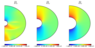Figure 5

In spite of the fact that our distributions of $\alpha_\circ$ and $\Omega$ do not depend on $r_c$ explicitly, model feels the inner core due to the imposed vacuum boundary condition at the inner core boundary. As we already mentioned above, this trick leads to the effective increase of the poloidal magnetic field generation at the boundary, clearly observed in Figure 5. We can associate this effect with the release of the latent heat, as well as with the enhanced viscous and Maxwell stresses at the inner core boundary in the more complex dynamo models.

We conclude that using this technique we provided the large-scale distributions of $\alpha_\circ$ and $\Omega$ in Eqs(3), took into account the growth of the inner core in the model, and obtained the dipole field configurations of the magnetic field for all $r_c$.

### The Random $\alpha$-effect

To the moment we did not discuss the time evolution of the magnetic fields, considering its spatial distributions only. As usually, the dipole magnetic fields in the mean-field model with geostrophic $\alpha_\circ$ and $\Omega$ are stationary. Increase of the energy sources leads to the switch from the dipole magnetic field to the multi-polar state, with already fluctuating, and may be reversing, dipole. Even if we find the transition region in the phase space, where the dipole's magnitude is still comparable to the other harmonics, the volume of this phase space will be very small, and it would be difficult to justify correspondence of exactly these parameters to the geodynamo regime. In other words, the sharper is the boundary between two states, the less is the probability of switch between these states.

Anyway, if we consider such fluctuating regimes, evolution of the magnetic dipole is very far from that one, observed in the geodynamo. The mean-field models demonstrate oscillations of the dipole, which resemble superposition of the periodic harmonics. On contrary, paleomagnetic observations point at existence of two attractors of the magnetic dipole at the geographic poles, and the quick transitions of the dipole between attractors: the reversals. Between the reversals there is the so-called regime in oscillations, where the dipole wanders around the pole, without change of the sign. The 3D dynamo models can indeed produce similar to observable behavior of the magnetic dipole, see, e.g., review [Roberts and King, 2013], but the mean-field models require additional modification.

The hint is that in 3D models there is the turbulence, which triggers the large-scale fluctuations. The mean-field dynamo models are too simple nonlinear systems to generate the small-scale fluctuations, and these fluctuations should be injected into the system by hand. It means that averaged quantities, used in the mean-field dynamo models, like $\alpha$-effect and differential rotation, must fluctuate.

Peter Hoyng was the first one who used these ideas in the solar dynamo [Hoyng, 1993], where minima of the solar activity are associated with the breaks of the dynamo cycle, caused by such fluctuations. Latter, stochastic $\alpha$-effect was used in the Galerkin's dynamo models [Sobko et al., 2012], where the geomagnetic dipole evolution was very similar to that one in the paleomagnetic records. The further analysis of the phase-space of 2D Parker's models, using the finite-differences [Reshetnyak, 2016], revealed some restrictions on the form of fluctuations of $\alpha$-effect. It looks attractive to use further the random $\alpha$-effect and explore how the growth of the inner core effects on the reversals statistic.

To introduce fluctuations of $\alpha$-effect we modify Eq. (4) as follows:

\begin{equation} \tag*{(6)}\displaystyle \alpha=C_\alpha\,{\alpha_\circ\left( 1+\epsilon \right) \over 1+E_m(r,\,\theta)}, \end{equation}

where $\epsilon$ is the random normal variable (the same for all the grid points) with zero mean value and standard deviation $\sigma$. The new fluctuation, introduced by $\epsilon$, was applied with the time step $\tau_f=0.1$. Then, after it, during the time $\tau_f$ $\alpha$ depended on the magnetic energy $E_m$ only.

We started from the state with the core size $r_c=0.35$ and the varied amplitudes of the noise: $\sigma=0.3,\,0.5,\,0.7,\,0.9$. The initial condition was taken from the solution, obtained in the previous Section. The first reversal was observed at $\sigma=0.5$. The further increase of $\sigma$ resulted in the gradual increase of the reversals number. For all $\sigma$ the magnetic field was dipole.

Then we performed similar simulations for the other two cases with $r_c=0.15$ and $0.25$. At the case with the small inner core, $r_c=0.15$, for all $\sigma$ the leading harmonic in the spatial spectrum was $l=3$ that corresponds to the anti-symmetric to the equator plane configuration, but with the smaller scale than for the dipole field, $l=1$.

We conclude that turbulent fluctuations in the liquid core lead to the decrease of the magnetic field scale at the surface of the core. Note that we considered only the dipole-like fluctuations of $\alpha$ in Eq. (5). Introduction of the independent fluctuations at the every grid point, immediately leads to the pike in the spatial spectrum at the small scales even for $r_c=0.35$, see [Reshetnyak, 2016]. This effect is concerned with the absence of the inverse cascade of the magnetic energy in Parker's equations Eq. (3).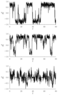Figure 6

The evolution of the magnetic dipole $g_1^0$ for $\sigma=0.7$ with varying $r_c$ is presented in Figure 6. For $r_c=0.25$ the amplitude of dipole fluctuations is slightly increased compared to the present time regime. However the number of the reversals changed only from 5 to 6, the number of the excursions increased in more extent. In the both cases the dipole component is the strongest one. Obviously, the length of the time interval is too short to insist on any additional significant distinctions of the cases with $r_c=0.25$ and $0.35$.

The third case with $r_c=0.15$ is very different from the previous two. As was already mentioned, the dominating harmonic is $l=3$. As regards to the dipole's behavior, it spends more time in the low latitudes with the short blowouts to the poles. This behavior is natural to the state, where the harmonic depends strongly on interaction with the large number of the other harmonics. The memory on the attractors at the poles is lost. Thus, fluctuations causes transition from the dipole magnetic field configuration to the octupole field at the small $r_c$. In other words, accordingly to this mean-field dynamo model, the dipole configuration of the magnetic field in the past, if it did exist, was unstable.

### Conclusions

We tried to present the consistent scenario of how information on the physical fields, obtained from the modern 3D dynamo simulations in the liquid core, could be included to the mean-field geodynamo model. This approach suggests that we distinguish the principal effects from the more complex models and then test them in the simpler ones. The correspondence of the obtained results to the 3D simulations and our expectations (based on some general knowledge on the system) let us to judge whether our suggestions were right or wrong.

Following this way we come to the quiet interesting phenomenon, concerned with the decrease of the magnetic field spatial scale in the past, caused by the small inner core. We emphasize that to test this effect in the 3D dynamo models, the low Ekman number regime is required.

Moreover, the majority of the dynamo models, which include sometimes the very different sources of the energy, nevertheless, have the same size of the inner core (equal to the present core's size). The latter obstacle lets to generate the dipole magnetic field regardless to the physical mechanism of convection.

On contrary, in the mean-field model, where the geostrophic balance is prescribed, only for the comparable size of the inner core to the modern one, the magnetic field's spectrum is dipole and stable to the turbulent perturbations. The decrease of the inner core's size from $r_c=0.35$ to $0.15$ leads to the dipole magnetic field as well, which is however already unstable.

It is worthy to note that dipole field generation is supported by the "volume" effects, like compressibility of the liquid, homogeneously distributed radioactive heating sources, and can be violated by the processes at the smaller scales: compositional convection at the stage of the small inner core, viscous and magnetic stresses at the inner core boundary.

So far the modern 3D dynamo models still lead to the quite contradictory scenarios, we believe that these suggestions will attract attention of the dynamo community and would be carefully checked with the higher level of accuracy, where it is possible.

#### Acknowledgment

The author acknowledges financial support from Russian Science Foundation, the grant No. 16-17-10097.

### References

Braginsky, S. I. (1975), Nearly axially symmetric model of the hydromagnetic dynamo of the Earth, I, Geomagnetism and Aeronomy, 15, p. 122–128.

Busse, F.-H. (1970), Thermal instabilities in rapidly rotating systems, Fluid Mech., 44, p. 441–460.

Busse, F.-H., R. D. Simitev (2008), Toroidal flux oscillation as possible cause of geomagnetic excursions and reversals, Phys. Earth Planet. Int., 168, p. 237–243, doi:10.1016/j.pepi.2008.06.007.

Glatzmaier, G., P. H. Roberts (1995), A three-dimensional convective dynamo solution with rotating and finitely conducting inner core and mantle, Phys. Earth Planet. Int., 91, p. 63–75.

Hoyng, P. (1993), Helicity fluctuations in mean field theory: an explanation for the variability of the solar cycle?, Astron. Astrophys., 272, p. 321–339.

Jouve, L., A. S. Brun, R. Arlt, A. Brandenburg, M. Dikpati, A. Bonanno, P. J. Käpylä, D. Moss, M. Rempel, P. Gilman, M. J. Korpi, A. G. Kosovichev (2008), A solar mean field dynamo benchmark, Astron. Astrophys., 483, p. 949–960, doi:10.1051/0004-6361:20078351.

Krause, F., K.-H. Rädler (1980), Mean-field magnetohydrodynamics and dynamo theory, 272 pp., Akademie-Verlag, Berlin.

Parker, E. N. (1995), Hydromagnetic dynamo models, Astrophys. J., 122, p. 293–314.

Reshetnyak, M. (2012), Effect of compressibility on the generation of hydrodynamic helicity in the Earth’s liquid core, Geomagnetism and Aeronomy, 52, no. 3, p. 398–402, doi:10.1134/S0016793212030139.

Reshetnyak, M. (2014), The mean-field dynamo model in geodynamo, Russ. J. Earth Sci., 14, p. ES2001, doi:10.2205/2014ES000539.

Reshetnyak, M. (2016), Parker's model in geodynamo, Magnetohydodynamics, 60, no. 2, p. 294–305.

Reshetnyak, M., V. Pavlov (2016), Evolution of the Dipole Geomagnetic Field. Observations and Models, Geomagnetism and Aeronomy, 56, no. 1, p. 110–124, doi:10.1134/S0016793215060122.

Roberts, P.-H. (1968), On the thermal instability of a rotating-fluid sphere containing heat sources, Phil. Trans. R. Soc., A263, p. 93–117.

Roberts, P.-H., E. M. King (2013), On the genesis of the Earth's magnetism, Rep. Prog. Phys., 76, no. 9, p. 096801–1, doi:10.1088/0034-4885/76/9/096801.

Sobko, G. S., V. N. Zadkov, D. D. Sokolov, V. I. Trukhin (2012), Geomagnetic reversals in a simple geodynamo model, Geomagnetism and Aeronomy, 52, no. 2, p. 254–260, doi:10.1134/S0016793212020144.

Tarduno, J. A., R. D. Cottrell, M. K. Watkeys, A. Hofmann, P. V. Doubrovine, E. A. Mamajek, D. Liu, D.G. Sibeck, L. P. Neukirch, Y. Usui (2010), Geodynamo, solar wind, and magnetopause 3.4 to 3.45 billion years ago, Science, 327, p. 1238–1240, doi:10.1126/science.1183445.

Wicht, J. (2002), Inner-core conductivity in numerical dynamo simulations, Phys. Earth Planet. Int., 132, p. 281–302, doi:10.1016/S0031-9201(02)00078-X.

Received 27 October 2016; accepted 23 November 2016; published 25 November 2016.Citation: Reshetnyak M. Yu. (2016), Growth of the inner core in the mean-field dynamo model, Russ. J. Earth Sci., 16, ES6003, doi:10.2205/2016ES000585.

Generated from LaTeX source by ELXpaper, v.1.5 software package.